upm  1.7.1 Sensor/Actuator repository for libmraa (v2.0.0)
H803X Class Reference

UPM API for the Veris H803X Energy Meter. More...

## Detailed Description

This module implements support for the Veris H8035 and H8036 Energy Meters.

The H8036 is similar to the H8035, but provides much more data.

The Enercept H8035/H8036 is an innovative three-phase networked (Modbus RTU) power transducer that combines electronics and high accuracy industrial grade CTs in a single package. The need for external electrical enclosures is eliminated, greatly reducing installation time and cost. Color-coordination between voltage leads and CTs makes phase matching easy. Additionally, these transducers automatically detect and compensate for phase reversal, eliminating the concern of CT load orientation. Up to 63 Transducers can be daisy-chained on a single RS-485 network.

This module was developed using libmodbus 3.1.2, and the H8035. The H8036 has not been tested. libmodbus 3.1.2 must be present for this module to build.

It was developed using an RS232->RS485 interface. You cannot use the built in MCU TTL UART pins for accessing this device – you must use a full Serial RS232->RS485 or USB-RS485 interface connected via USB.

string defaultDev = "/dev/ttyUSB0";
// if an argument was specified, use it as the device instead
if (argc > 1)
defaultDev = string(argv);
cout << "Using device " << defaultDev << endl;
cout << "Initializing..." << endl;
// Instantiate an H803X instance, using MODBUS slave address 1, and
// default comm parameters (9600, 8, N, 2)
upm::H803X sensor(defaultDev, 1);
// output the Slave ID string
cout << "Slave ID: " << sensor.getSlaveID() << endl;
cout << endl;
// update and print available values every second
while (shouldRun) {
// update our values from the sensor
sensor.update();
// H8035 / H8036
cout << "Consumption (kWh): " << sensor.getConsumption() << endl;
cout << "Real Power (kW): " << sensor.getRealPower() << endl;
if (sensor.isH8036()) {
// The H8036 has much more data available...
cout << "Reactive Power (kVAR): " << sensor.getReactivePower() << endl;
cout << "Apparent Power (kVA): " << sensor.getApparentPower() << endl;
cout << "Power Factor: " << sensor.getPowerFactor() << endl;
cout << "Volts Line to Line: " << sensor.getVoltsLineToLine() << endl;
cout << "Volts Line to Neutral: " << sensor.getVoltsLineToNeutral() << endl;
cout << "Current: " << sensor.getCurrent() << endl;
cout << "Real Power Phase A (kW): " << sensor.getRealPowerPhaseA() << endl;
cout << "Real Power Phase B (kW): " << sensor.getRealPowerPhaseB() << endl;
cout << "Real Power Phase C (kW): " << sensor.getRealPowerPhaseC() << endl;
cout << "Power Factor Phase A: " << sensor.getPowerFactorPhaseA() << endl;
cout << "Power Factor Phase B: " << sensor.getPowerFactorPhaseB() << endl;
cout << "Power Factor Phase C: " << sensor.getPowerFactorPhaseC() << endl;
cout << "Volts Phase A to B: " << sensor.getVoltsPhaseAToB() << endl;
cout << "Volts Phase B to C: " << sensor.getVoltsPhaseBToC() << endl;
cout << "Volts Phase A to C: " << sensor.getVoltsPhaseAToC() << endl;
cout << "Volts Phase A to Neutral: " << sensor.getVoltsPhaseAToNeutral() << endl;
cout << "Volts Phase B to Neutral: " << sensor.getVoltsPhaseBToNeutral() << endl;
cout << "Volts Phase C to Neutral: " << sensor.getVoltsPhaseCToNeutral() << endl;
cout << "Current Phase A: " << sensor.getCurrentPhaseA() << endl;
cout << "Current Phase B: " << sensor.getCurrentPhaseB() << endl;
cout << "Current Phase C: " << sensor.getCurrentPhaseC() << endl;
cout << "Avg Real Power (kW): " << sensor.getAvgRealPower() << endl;
cout << "Min Real Power (kW): " << sensor.getMinRealPower() << endl;
cout << "Max Real Power (kW): " << sensor.getMaxRealPower() << endl;
}
cout << endl;
upm_delay(2);
}
cout << "Exiting..." << endl;

## Public Types

enum  HOLDING_REGS_T {
HOLDING_CONSUMPTION_KWH_INT_L = 0, HOLDING_CONSUMPTION_KWH_INT_H = 1, HOLDING_CONSUMPTION_KWH = 258, HOLDING_REAL_POWER_KW = 260,
HOLDING_REACTIVE_POWER_KVAR = 262, HOLDING_APPARENT_POWER_KVA = 264, HOLDING_POWER_FACTOR = 266, HOLDING_VOLTS_LINE_TO_LINE = 268,
HOLDING_VOLTS_LINE_TO_NEUTRAL = 270, HOLDING_CURRENT = 272, HOLDING_REAL_POWER_PHASE_A_KWH = 274, HOLDING_REAL_POWER_PHASE_B_KWH = 276,
HOLDING_REAL_POWER_PHASE_C_KWH = 278, HOLDING_POWER_FACTOR_PHASE_A = 280, HOLDING_POWER_FACTOR_PHASE_B = 282, HOLDING_POWER_FACTOR_PHASE_C = 284,
HOLDING_VOLTS_PHASE_AB = 286, HOLDING_VOLTS_PHASE_BC = 288, HOLDING_VOLTS_PHASE_AC = 290, HOLDING_VOLTS_PHASE_AN = 292,
HOLDING_VOLTS_PHASE_BN = 294, HOLDING_VOLTS_PHASE_CN = 296, HOLDING_CURRENT_PHASE_A = 298, HOLDING_CURRENT_PHASE_B = 300,
HOLDING_CURRENT_PHASE_C = 302, HOLDING_AVG_REAL_POWER_KW = 304, HOLDING_MIN_REAL_POWER_KW = 306, HOLDING_MAX_REAL_POWER_KW = 308
}

enum  MULTIPLIERS_T {
MULT_100A = 128, MULT_300A_400A = 32, MULT_800A = 16, MULT_1600A = 8,
MULT_2400A = 4
}

## Public Member Functions

H803X (std::string device, int address, int baud=9600, int bits=8, char parity='N', int stopBits=2)

~H803X ()

void update ()

std::string getSlaveID ()

void presetConsumption (float value, MULTIPLIERS_T multiplier)

float getConsumption ()

float getRealPower ()

float getReactivePower ()

float getApparentPower ()

float getPowerFactor ()

float getVoltsLineToLine ()

float getVoltsLineToNeutral ()

float getCurrent ()

float getRealPowerPhaseA ()

float getRealPowerPhaseB ()

float getRealPowerPhaseC ()

float getPowerFactorPhaseA ()

float getPowerFactorPhaseB ()

float getPowerFactorPhaseC ()

float getVoltsPhaseAToB ()

float getVoltsPhaseBToC ()

float getVoltsPhaseAToC ()

float getVoltsPhaseAToNeutral ()

float getVoltsPhaseBToNeutral ()

float getVoltsPhaseCToNeutral ()

float getCurrentPhaseA ()

float getCurrentPhaseB ()

float getCurrentPhaseC ()

float getAvgRealPower ()

float getMinRealPower ()

float getMaxRealPower ()

void setDebug (bool enable)

bool isH8036 ()

## Protected Member Functions

int readHoldingRegs (HOLDING_REGS_T reg, int len, uint16_t *buf)

void writeHoldingReg (HOLDING_REGS_T reg, int value)

void clearData ()

void testH8036 ()

## Protected Attributes

modbus_t * m_mbContext

bool m_isH8036

## Constructor & Destructor Documentation

 H803X ( std::string device, int address, int baud = `9600`, int bits = `8`, char parity = `'N'`, int stopBits = `2` )

H803X constructor

Parameters
 device Path to the serial device address The MODBUS slave address baud The baudrate of the device. Default: 9600 bits The number of bits per byte. Default: 8 parity The parity of the connection, 'N' for None, 'E' for Even, 'O' for Odd. Default: 'N' stopBits The number of stop bits. Default: 2

Here is the call graph for this function: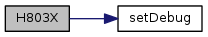~H803X ( )

H803X Destructor

## Member Function Documentation

 void update ( void )

Read current values from the sensor and update internal stored values. This method must be called prior to querying any values.

Here is the call graph for this function: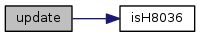string getSlaveID ( )

Return a string corresponding the the device's MODBUS slave ID.

Returns
string represnting the MODBUS slave ID

Set a new MODBUS slave address. This is useful if you have multiple H803X devices on a single bus. When this method is called, the current stored data is cleared, and a new attempt is made to determine whether the target device is an H8035 or H8036.

Parameters
 addr The new slave address to set
 void presetConsumption ( float value, MULTIPLIERS_T multiplier )

Preset the kWh accumulated Consumption registers to a predefined value. This is generally not advised, but is provided for those installations that might require it. The multiplier depends on the current range of your device. Be sure to select the right multiplier for your devices' supported current capacity.

Parameters
 value The desired value for the consumption accumulator registers in kWh. multiplier The correct MULTIPLIERS_T value for your device.
 float getConsumption ( )
inline

Return the accumulated consumption value, in kWh. update() must have been called prior to calling this method.

Returns
The accumulated consumption.
 float getRealPower ( )
inline

Return the real power value in kW. update() must have been called prior to calling this method.

Returns
The real power value in kW.
 float getReactivePower ( )
inline

Return the reactive power value in kVAR (kilo-volt Amperes Reactive). update() must have been called prior to calling this method.

Returns
The reactive power value in kVAR.
 float getApparentPower ( )
inline

Return the apparent power value in kVA. update() must have been called prior to calling this method.

Returns
The apparent power value in kVA.
 float getPowerFactor ( )
inline

Return the power factor value. update() must have been called prior to calling this method.

Returns
The power factor.
 float getVoltsLineToLine ( )
inline

Return the voltage line to line value. update() must have been called prior to calling this method.

Returns
The voltage, line to line.
 float getVoltsLineToNeutral ( )
inline

Return the voltage line to neutral. update() must have been called prior to calling this method.

Returns
The voltage, line to neutral.
 float getCurrent ( )
inline

Return the current value in amps. update() must have been called prior to calling this method.

Returns
The current value in amps.
 float getRealPowerPhaseA ( )
inline

Return the real power for phase A. update() must have been called prior to calling this method.

Returns
The real power for phase A.
 float getRealPowerPhaseB ( )
inline

Return the real power for phase B. update() must have been called prior to calling this method.

Returns
The real power for phase B.
 float getRealPowerPhaseC ( )
inline

Return the real power for phase C. update() must have been called prior to calling this method.

Returns
The real power for phase C.
 float getPowerFactorPhaseA ( )
inline

Return the power factor for phase A. update() must have been called prior to calling this method.

Returns
The power factor for phase A.
 float getPowerFactorPhaseB ( )
inline

Return the power factor for phase B. update() must have been called prior to calling this method.

Returns
The power factor for phase B.
 float getPowerFactorPhaseC ( )
inline

Return the power factor for phase C. update() must have been called prior to calling this method.

Returns
The power factor for phase C.
 float getVoltsPhaseAToB ( )
inline

Return the voltage for phase A to B. update() must have been called prior to calling this method.

Returns
The voltage for phase A to B.
 float getVoltsPhaseBToC ( )
inline

Return the voltage for phase B to C. update() must have been called prior to calling this method.

Returns
The voltage for phase B to C.
 float getVoltsPhaseAToC ( )
inline

Return the voltage for phase A to B. update() must have been called prior to calling this method.

Returns
The voltage for phase A to B.
 float getVoltsPhaseAToNeutral ( )
inline

Return the voltage for phase A to neutral. update() must have been called prior to calling this method.

Returns
The voltage for phase A to neutral.
 float getVoltsPhaseBToNeutral ( )
inline

Return the voltage for phase B to neutral. update() must have been called prior to calling this method.

Returns
The voltage for phase B to neutral.
 float getVoltsPhaseCToNeutral ( )
inline

Return the voltage for phase C to neutral. update() must have been called prior to calling this method.

Returns
The voltage for phase C to neutral.
 float getCurrentPhaseA ( )
inline

Return the current for phase A. update() must have been called prior to calling this method.

Returns
The current for phase A.
 float getCurrentPhaseB ( )
inline

Return the current for phase B. update() must have been called prior to calling this method.

Returns
The current for phase B.
 float getCurrentPhaseC ( )
inline

Return the current for phase C. update() must have been called prior to calling this method.

Returns
The current for phase C.
 float getAvgRealPower ( )
inline

Return the average real power. update() must have been called prior to calling this method.

Returns
The average real power.
 float getMinRealPower ( )
inline

Return the minimum real power. update() must have been called prior to calling this method.

Returns
The minimum real power.
 float getMaxRealPower ( )
inline

Return the maximum real power. update() must have been called prior to calling this method.

Returns
The maximum real power.

Here is the call graph for this function: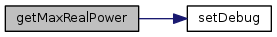void setDebug ( bool enable )

Enable or disable debugging output. This primarily enables and disables libmodbus debugging output.

Parameters
 enable true to enable debugging, false otherwise

Here is the caller graph for this function: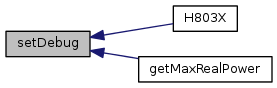bool isH8036 ( )
inline

Indicate whether the connected device is an H8035 or an H8036. The H8036 provides many more data registers.

Returns
true if we are using an H8036, false otherwise.

Here is the caller graph for this function: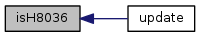The documentation for this class was generated from the following files: